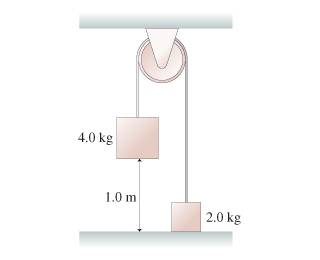# Rotation of a Rigid Body

## Homework Statement

The two blocks in the figure are connected by a massless rope that passes over a pulley. The pulley is 14cm in diameter and has a mass of 2.4 kg. As the pulley turns, friction at the axle exerts a torque of magnitude 0.54 Nm .## Homework Equations

so m1 = 4.0kg
m2= 2.0 kg
M = 2.4kg
r= 0.07m
Tf (torque due to friction) = 0.54 Nm
ƩFy (for mass 1)= T2 - m1*g
m1*ay1 +m1*g= T2 so T2 = m1(ay1+g) this becomes T2= m1(ay + g)

ƩFy (for mass 2)= T1 - m2*g
m1*ay2 + m2*g = T1 so T1 = m2(ay2 + g)
but since ay1 = -ay2 = ay this becomes T1 = m2(g - ay)

Ʃτ= T2*R - T1*R - Tf

## The Attempt at a Solution

Using what i put up there i get the following formula

τnet = R(T2 - T1) - Tf = R(m1(ay+g) - m2(g-ay)) - Tf .... equation 1
since τ= Iα, I= 1/2 MR^2 and since α= -ay/R

equation 1 becomes..

1/2MR^2 * (-ay)R = R(m1(ay+g) - m2(g-ay)) - Tf ..... solving for ay with the given data i found ay=-1.65 and thus using the kinematic equation i found Δt= 1.55s but this is wrong.. :( i would appreciate if anyone points out my error or mistake, thanks
EDIT: sorry about this, they are asking us to find the time it takes for mass 1 to hit the floor, starting at rest.

Last edited:

Redbelly98
Staff Emeritus
Homework Helper
Well, you never said what the actual question is. Looks like you are to figure out how long it takes the 4 kg mass to hit the floor? And everything is initially at rest?

I agree with the 1.65 m/s2 acceleration, so your mistake is probably in applying the kinematic equations. Can you show that work?

p.s. It's kind of confusing that you chose T2 for the rope holding m1, and T1 for the rope holding m2. Nonetheless you did find the correct acceleration.

thanks! and sorry about that! Yes it is kinda confusing but im happy to know that someone else got the same acceleration. Well, I tried applying the kinematic formula:

yf = yi + Vi*t + 1/2 a t^2 ... t = delta t... and i chose the initial point to be 0, so the final point would be -2m since it goes down... also, the initial velocity is zero since it starts from rest... so..

-2.0m = 0 + 0 * t + 1/2 * (-1.65m/s^2) * t^2
thus...

-2.0 m / -1.65 m/s^2 = t^2.... applying square root i get 1.1s......which seems to be the right answer... what the hell was i doing wrong?? oh wow i think i was not dividing properly... lol so all this time i actually had the right answer before my eyes but never realized that i was making a silly mistake... my mistake was applying the kinematic formula.. well thanks for your help have a nice day :)

Filip Larsen
Gold Member
[STRIKE]I get an acceleration of around 3.7 m/s and around 0.7 s.[/STRIKE] The only thing I can spot is that you multiply with R on the left-hand side of you last equation in your first post instead of dividing, but that just looks like a typo.

Edit: Just for the record, I made a sign error. Doing it properly I too get 1.7 m/s2 and 1.1 s.

Last edited:
Redbelly98
Staff Emeritus
I get 1.1 s as well.Be careful, it actually drops 1.0 m in 1.1 s, not 2.0 m.